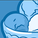# New to Qlik Sense

If you’re new to Qlik Sense, start with this Discussion Board and get up-to-speed quickly.

Announcements
Join us for a live Q&A! September 21, 10 AM ET - Onboarding Fast in Qlik Sense SaaS! REGISTER
cancel
Showing results for
Did you mean:Creator III

## help with measure set analysis

Hello all,

I'm looking to calculate a measure: a person who has done at least two checks, the last being in the last 36 months. Also the interval between the last and the previous of last time should be less than 12 months.

I did

Count(DISTINCT {<
[person ID] = {"=Count(DISTINCT [date]) >= 2 and Max([date]) >= AddMonths(Today(), -36) and max([date])<=Today() and round((max([date])-max([date],2))/365)<='1'"}
>}
[person ID])

with count(distinct date)>=2 I mean to say that there are at least two  diferent control dates .
but although there is no code error, the results i want are not correct. So i was wondering If you could help?

1 Solution

Accepted SolutionsSpecialist

I use it to satisfy the criteria of the interval between the most recent date and the one prior should be less than 12 months.

max([date],2) represents the data previous to the most recent.  The Addmonths function adds 12 months to the date previous to the most recent date.  So, if that value is greater than the max([date]), I know the checks came within 12 months of each other.

8 RepliesSpecialist

Your expression looks reasonable.  I'm wondering if your calculation to determine the interval between the last date and the one before it is causing the issue:

Count(DISTINCT {<
[person ID] = {"=Count(DISTINCT [date]) >= 2 and Max([date]) >= AddMonths(Today(), -36) and max([date])<=Today() and max([date])<=Addmonths(max([date],2),12)"}>}
[person ID])Creator III
Author

Can you explain further what this Addmonths(max([date],2),12)   did?  @GaryGilesSpecialist

I use it to satisfy the criteria of the interval between the most recent date and the one prior should be less than 12 months.

max([date],2) represents the data previous to the most recent.  The Addmonths function adds 12 months to the date previous to the most recent date.  So, if that value is greater than the max([date]), I know the checks came within 12 months of each other.Creator III
Author

Thank you Gary! @GaryGiles  😁👏

I have another one which confuses me. Now I have to calculate the person who: has had checks done before BUT has only one check in the last 2 months. Also the interval between the last and the one before the last one is greater than 12 months.

I did :

Count(DISTINCT {
[person ID]= {"= Count(DISTINCT [date]) >= 2  and ( count(Distinct [date] =1 and Max([date]) >= AddMonths(Today(), -2) ) and max([date])<=Today() and max([date])<=Addmonths(max([date],2),12)"}
>}
[person ID])

but i'm not sure... What would you do in order to include both the fact that there are more than one date checks but only one in the last  2 months?Specialist

I think you are looking for something like this:  the 2nd count limits it to only customers who have had only 1 check in the last 2 months.

Count(DISTINCT {
[person ID]= {"= Count(DISTINCT [date]) >= 2  and count({\$<date={[>=\$(=AddMonths(Today(),-2))]}>} Distinct date)=1 and max([date])<=Today() and max([date])<=Addmonths(max([date],2),12) and "}
>}
[person ID])Creator III
Author

Count(DISTINCT {
[person ID]= {"= Count(DISTINCT [date]) >= 2  and count({\$<date={[>=\$(=AddMonths(Today(),-2))]}>} Distinct date)=1 and max([date])<=Today() and max([date])<=Addmonths(max([date],2),12) and "}
>}
[person ID])

I removed the "and" in red and Qlik says a "}" is expected. Maybe at the part in blue?

Trying to decipher this 😁

@GaryGiles thanks for the help, appreciated.Specialist

It's a little hard to decipher without the actual data model.  Try this:

Count({\$<[person ID]= {"=(Count(DISTINCT [date]) >= 2  and count({\$<date={[>=\$(=AddMonths(Today(),-2))]}>} Distinct date)=1 and max([date])<=Today() and max([date])<=Addmonths(max([date],2),12) )"}
>} DISTINCT  [person ID])Creator III
Author

following the same thinking, shouldn't someone who has more than one date checks ever but none in the last 12 months be like:

Count({\$< [person ID]= {"=(Count(DISTINCT [date]) >= 1 and count({\$<[date]={[>=\$(=AddMonths(Today(),-12))]}>} Distinct [date])=0 and max([date])<=Today() "}
>} DISTINCT [person ID])

?

It isn't . I'm doing trials right now to see if I can do some on my own🤔@GaryGilesTags
Community Browser